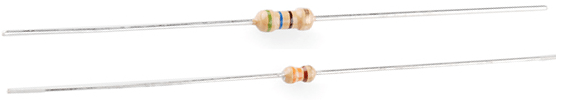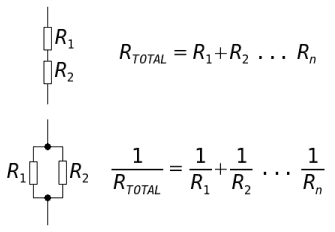Objectives:

• To be able to calculate the total resistance of two or more resistors in series using$R_{T} = R_{1} + R_{2} + R_{3} + ...$
• To be able to calculate the total resistance of two or more resistors in parallel using$\frac{1}{R_{T}} = \frac{1}{R_{1}} + \frac{1}{R_{2}} + \frac{1}{R_{3}} + ...$
• To be able to analyse of circuits with components, including both series and parallelResistors are components that increase the resistance along a path of a circuit. They can be made of a number of different materials including types of alloys, carbon or a material coated in ceramic.

A metal wire has a resistance, as discussed in previous sessions – link, there are ions held in a fixed lattice in which the flow of electrons needs to pass by.If the length of the wire were to double, there would be twice as many ions to pass and so there would be twice as much resistance.

Resistors in Series

Resistors placed in series increase the total resistance of the circuit in the same way that increasing the length of the wire increases the total resistance.Take this circuit, if each resistor had a resistance of$10 \ \Omega$  then the total resistance of this circuit would be$30 \ \Omega$ .

If each resistor has a different value of resistance e.g.$R_{1} = 10 \ \Omega$$R_{2} = 20 \ \Omega$  and$R_{3} = 30 \ \Omega$ , the total resistance would be the sum of all three:$R_{T} = 10 + 20 + 30 = 60 \ \Omega$

The following equation can be used to calculate the total resistance of a series of resistors in series:$R_{T} = R_{1} + R_{2} + R_{3} + ...$

This equation can be proved to work experimentally but it can also be derived by using Kirchhoff’s two laws, try it for yourself, a full derivation can be found here – Deriving the Equation for Resistors in Series.

Resistors in Parallel

Resistors placed in parallel decrease the total resistance of the circuit. The reason for this is that there are more paths for the charged particles to travel down. Take this circuit for instance;• If we initially only had resistor$R_{1}$  in place and this had an an extremely high resistance, say$R_{1} = \infty \ \Omega$ , then the electrons would struggle to flow through it and hence around the circuit at all.
• If resistor 2 was then inserted into the position shown and has a low resistance, say$R_{2} = 10 \ \Omega$ , then the electrons have the ability to travel down this path instead and hence can allow a current around the circuit.
• Inserting the second resistor in parallel has allowed more current to flow and thus has decreased the overall resistance of the circuit.

Take the following circuit;The lowest value resistor here is$10 \ \omega$ , the total resistance of the three resistors must be lower than this value due to the points outlined above.

The following equation can be used to calculate the total resistance of a set of resistors in parallel:$\frac{1}{R_{T}} = \frac{1}{R_{1}} + \frac{1}{R_{2}} + \frac{1}{R_{3}} + ...$

This equation can be proved to work experimentally but it can also be derived by using Kirchhoff’s two laws, try it for yourself, a full derivation can be found here – Deriving the Equation for Resistors in Parallel.

The total resistance of this circuit is therefore calculated as shown;$\frac{1}{R_{T}} = \frac{1}{10} + \frac{1}{20} + \frac{1}{10}$$\frac{1}{R_{T}} = \frac{2}{20} + \frac{1}{20} + \frac{2}{20}$$\frac{1}{R_{T}} = \frac{5}{20}$

For those of you who are rebellious and decide not to show your workings can easily fall into the trap of now writing$R_{T} = 0.25 \ \Omega$  – this is INCORRECT. the equation we have at this stage is the inverse of$R_{T}$  and so the answers needs to be flipped;$\frac{1}{R_{T}} = \frac{5}{20}$$\frac{R_{T}}{1} = \frac{20}{5}$$R_{T} = 4 \ \Omega$Questions:

Three resistors, two with a resistance$25 \ \Omega$  each and one with$50 \ \Omega$  are connected together in a circuit in the following combinations. Work out the total resistance for each case:

i) all connected in series together.
ii) all connected in parallel to one another
iii) the two$25 \ \Omega$  resistors are connected in series together but in parallel to the$50 \ \Omega$  resistor.
iv) the two$25 \ \Omega$  resistors are connected in parallel to one another, and this combination is connected in series to the$50 \ \Omega$  resistor.

Hint: Draw each circuit and try calculating the overall resistance of certain parts separately.

If you do not obtain the following answers try again until you do;

i)$100 \ \Omega$
ii)$10 \ \Omega$
iii)$25 \ \Omega$
iv)$62.5 \ \Omega$  (or$63 \ \Omega$  to 2.s.f)

How many resistor combinations can be made using three identical resistors each with a resistance of$1 \ k\Omega$ ? What are each of the values of the total resistance for each combination?

Repeat the question but with four$1 \ k\Omega$  resistors.